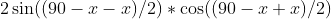# Trigonometry : Complete a Proof Using Sums, Differences, or Products of Sines and Cosines

## Example Questions

### Example Question #1 : Complete A Proof Using Sums, Differences, Or Products Of Sines And Cosines

True or false: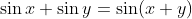.

False

Cannot be determined

True

False

Explanation:

The sum of sines is given by the formula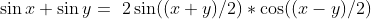.

### Example Question #2 : Complete A Proof Using Sums, Differences, Or Products Of Sines And Cosines

True or false: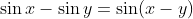.

False

Cannot be determined

True

False

Explanation:

The difference of sines is given by the formula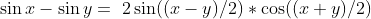.

### Example Question #3 : Complete A Proof Using Sums, Differences, Or Products Of Sines And Cosines

True or false: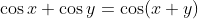.

True

Cannot be determined

False

False

Explanation:

The sum of cosines is given by the formula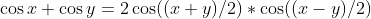.

### Example Question #1 : Complete A Proof Using Sums, Differences, Or Products Of Sines And Cosines

True or false: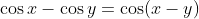.

Cannot be determined

True

False

False

Explanation:

The difference of cosines is given by the formula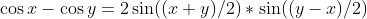.

### Example Question #5 : Complete A Proof Using Sums, Differences, Or Products Of Sines And Cosines

Which of the following correctly demonstrates the compound angle formula?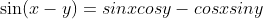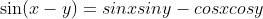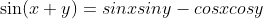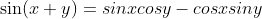Explanation:

The compound angle formula for sines states that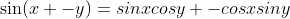.

### Example Question #6 : Complete A Proof Using Sums, Differences, Or Products Of Sines And Cosines

Which of the following correctly demonstrates the compound angle formula?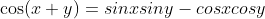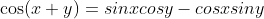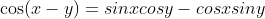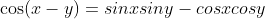Explanation:

The compound angle formula for cosines states that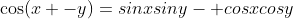.

### Example Question #7 : Complete A Proof Using Sums, Differences, Or Products Of Sines And Cosines

Simplify by applying the compound angle formula: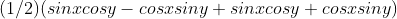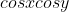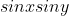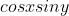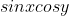Explanation:

Using the compound angle formula, we can rewrite each half of the non-coefficient terms in the given expression. Given thatand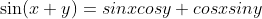, substitution yields the following: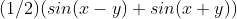This is the formula for the product of sine and cosine,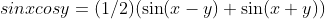.

### Example Question #8 : Complete A Proof Using Sums, Differences, Or Products Of Sines And Cosines

Simplify by applying the compound angle formula: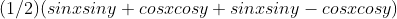Explanation:

Using the compound angle formula, we can rewrite each half of the non-coefficient terms in the given expression. Given that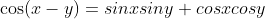and, substitution yields the following: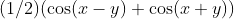This is the formula for the product of two cosines,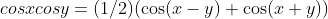.

### Example Question #9 : Complete A Proof Using Sums, Differences, Or Products Of Sines And Cosines

Using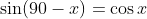and the formula for the sum of two sines, rewrite the sum of cosine and sine: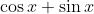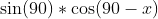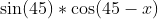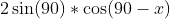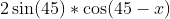Explanation:

Substitute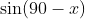for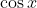: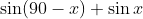Apply the formula for the sum of two sines,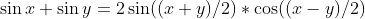: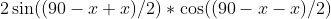### Example Question #10 : Complete A Proof Using Sums, Differences, Or Products Of Sines And Cosines

Usingand the formula for the difference of two sines, rewrite the difference of cosine and sine: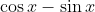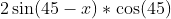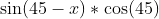Explanation:

Substitutefor: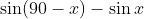Apply the formula for the difference of two sines,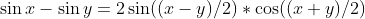.